Data Manipulation   «Prev  Next»
 Lesson 3 Character functions: CONCAT, SUBSTR, LENGTH, INSTR Objective Describe the use of the character functions CONCAT, SUBSTR, LENGTH, and INSTR.

# Oracle Character Functions CONCAT, SUBSTR, LENGTH, INSTR

## Character Functions: CONCAT, SUBSTR, LENGTH, INSTR

Four commonly used string functions are
1. concatenate,
2. substring,
3. length, and
4. instring.

## CONCAT or ||

The concatenation function uses different syntax for different programming languages and software. For Oracle, you have two choices:
1. Use two vertical bars (||) to connect the two columns you wish to combine. This is by far the most commonly used variation and you can easily concatenate three columns (or expressions or literal values) together by repeating the vertical bars.
2. Use the `CONCAT(a,b)` function. This function concatenates the first column with the second column. To add a third column, you must add another `CONCAT(a,b)` around the first one.
Here is an example (using the course project) of the first, most commonly used, form of concatenation:

```SELECT CITY || ', ' || STATE || '  ' || ZIP
FROM CUSTOMER;
```

The results look like this:
```CITY||','||STATE||''||ZIP
-----------------------------------
Omaha, NE  98765
Makena, HI  99800
Hollywood, CA  10293
Kihei, HI  39873
```

### Oracle LENGTH

This simple function tells you the length (in number of characters) of the data in a column, expression, or literal. In the case of `VARCHAR2` datatype columns, the defined length of the column is often higher than the actual data stored in each row's column. Use the Oracle length function to count the characters.
If a field contains no data (is null) then its length is null.

### Oracle INSTR

The instring function returns a number – the number of the position within the field of the character you specify. The SlideShow below shows the syntax and examples for the `INSTR` function.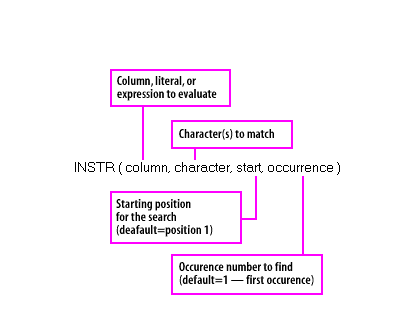1) The syntax of the INSTR function, including parameters. If the character or characters are not found, the INSTR function returns zero.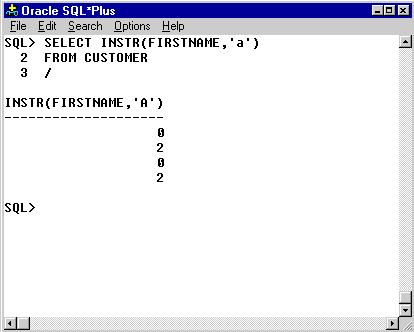2) This example shows how to search for the letter "a" in the LASTNAME column of the CUSTOMER table. The searching used in this function is case-sensitive. In this case, the default starting position and occurrence number were used because these two parameters were omitted.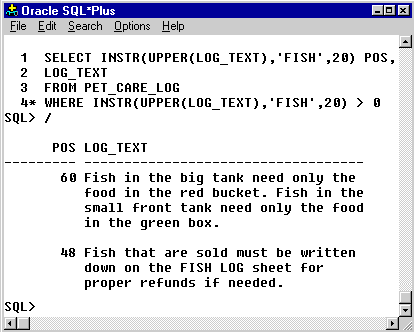3) Add a position number as the third parameter to start the search for the character at some point within the field. For example, if you want to find the word "the" in the LOG_TEXT column, but only in the 20th character or beyond, the query would look like the one shown here. The identical function was added to the WHERE clause to limit the rows returned to those that match the INSTR function. SELECT INSTR(UPPER(LOG_TEXT), 'FISH', 20) POS, LOG_TEXT FROM PET_CARE_LOG WHERE INSTR(UPPER(LOG_TEXT), 'FISH', 20) > 0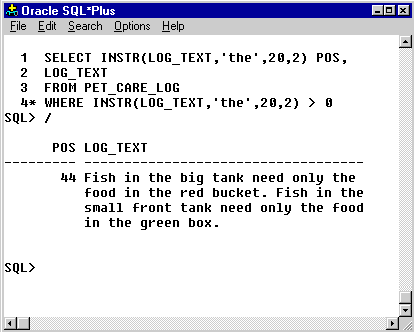4) You can find the second, third, or other occurrence of the character by adding a third parameter to the function. For example, if you wish to find the second occurrence of the word "the" in the LOG_TEXT column, the query would be revised to include both the starting position (20) and the occurence number (2) as shown here.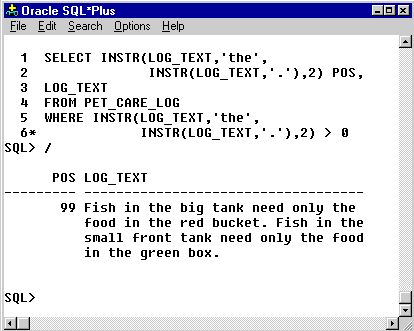5) You can use an INSTR function to set the starting position of another INSTR function. In this example, we have replaced the starting position (20) with an INSTR function that looks for period (.). In effect, we are setting the starting position at the end fo the first sentence in the text.

Instr Function Syntax

### Oracle SUBSTR

The substring function, which returns part of the column, is written like as follows:
```SUBSTR(col1, start, length)
```

You can combine the substring function with the instring function and create a useful tool. Here is an example, using the Pet Store schema.
Let us say you want to retrieve only the first sentence from each Pet Log entry. You can use the substring function to return a portion of the data, but the exact length of the sentence varies with each entry. By using the instring function, you can locate the first period (.) found in the data. This is where the sentence ends. Add one to this number, and you can retrieve the complete sentence, including the period. The diagram below shows the SQL query that does it.

The first command you see in the figure is a SQL*Plus column formatting command. It lets me show you the entire contents of the column in a narrow window. See the module titled "The Oracle SQL*Plus environment" for more information on SQL*Plus commands. In the next lesson, you will learn use of the character functions `UPPER`, `INITCAP`, `RTRIM`, and `SOUNDEX`.